# Invariant imbedding

A method of converting certain two-point boundary value problems to initial value problems. It is an outgrowth of the method of invariance, introduced by V.A. Ambarzumyan (V.A. Ambartsumyan) (cf. [a1]) and used so successfully by S. Chandrasekhar in radiative transfer problems (cf. [a2]). Invariant imbedding is closely related to the sweep method (cf. also Double-sweep method; Shooting method).

Consider the system of linear ordinary scalar differential equations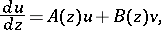(a1)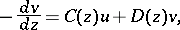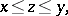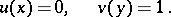(Whenandare non-negative, (a1) may be considered as describing a flow of right-moving particles,, and left-moving particles,, with unit input atand no input at. This interpretation is often helpful but is by no means necessary.) One asks for. (In the flow model,is a reflection coefficient.)

Write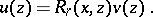(a2)

Substitution of (a2) into (a1) yields, formally,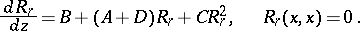(a3)

This Riccati equation may, under certain conditions, be integrated fromtoto yield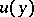. If one defines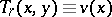, then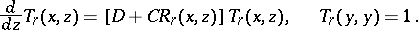(a4)

If the boundary conditions in (a1) are simply switched, analogous functions,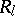and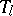, can be defined. Like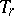, they satisfy linear initial value equations. Only (a3) is non-linear (cf. [a3]).

The approach becomes much more valuable when the boundary conditions in (a1) are replaced by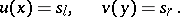(a5)

Then, for any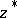,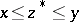,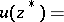(a6)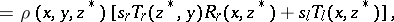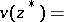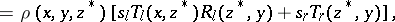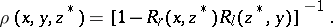There are many extensions and generalizations of these ideas. A few are:

a) Equation (a1) may be replaced by a system withan-vector anda-vector.

b) Inhomogeneous terms may be appended to (a1).

c) Conditions (a5) may be replaced by mixed boundary conditions.

d) If (a3) fails to have a solution for all,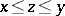, various devices may be employed to cross the singularity.

In all cases the function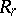plays the crucial role and is the only function obtained by solving a non-linear equation. All other problems are linear. (Note: the theory may also be built around, which then satisfies a Riccati equation.)

Invariant imbedding concepts have been employed to investigate problems in transport theory (cf. also Transport equations, numerical methods), wave propagation, difference equations, integral equations, quantum mechanics, and many other areas of mathematics and theoretical physics.